7 Fixed Points PS Fixed Points Roadmap Representing

• Slides: 307. Fixed PointsPS — Fixed Points Roadmap > > > Representing Numbers Recursion and the Fixed-Point Combinator The typed lambda calculus The polymorphic lambda calculus Other calculi © O. Nierstrasz 7. 2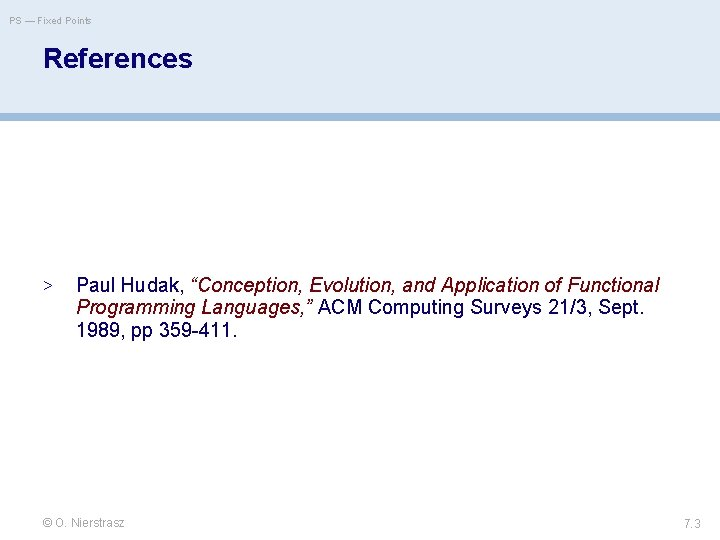PS — Fixed Points References > Paul Hudak, “Conception, Evolution, and Application of Functional Programming Languages, ” ACM Computing Surveys 21/3, Sept. 1989, pp 359 -411. © O. Nierstrasz 7. 3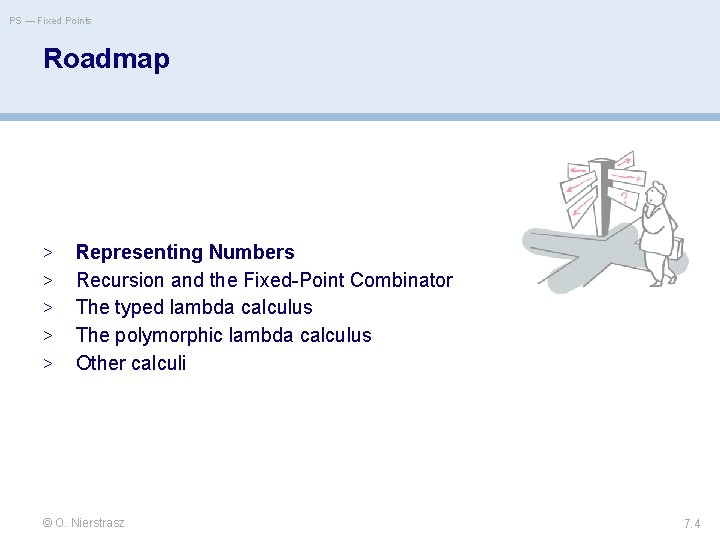PS — Fixed Points Roadmap > > > Representing Numbers Recursion and the Fixed-Point Combinator The typed lambda calculus The polymorphic lambda calculus Other calculi © O. Nierstrasz 7. 4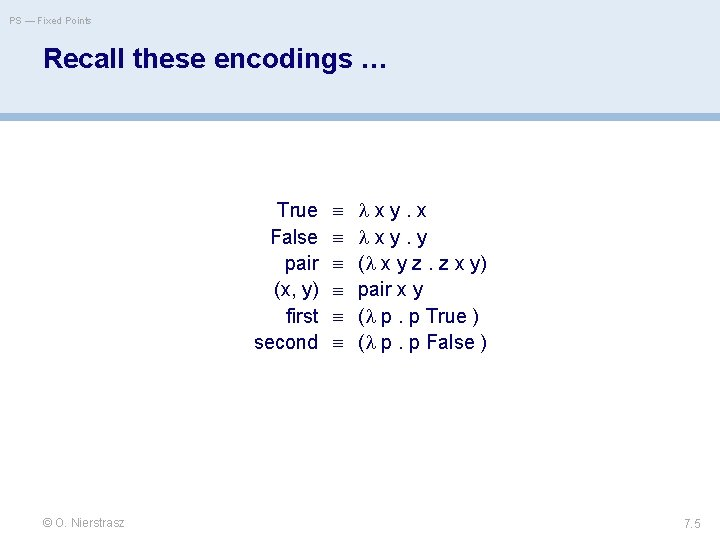PS — Fixed Points Recall these encodings … True False pair (x, y) first second © O. Nierstrasz x y. x x y. y ( x y z. z x y) pair x y ( p. p True ) ( p. p False ) 7. 5PS — Fixed Points Representing Numbers There is a “standard encoding” of natural numbers into the lambda calculus: Define: 0 ( x. x ) succ ( n. (False, n) ) then: © O. Nierstrasz 1 succ 0 (False, 0) 2 succ 1 (False, 1) 3 succ 2 (False, 2) 4 succ 3 (False, 3) 7. 6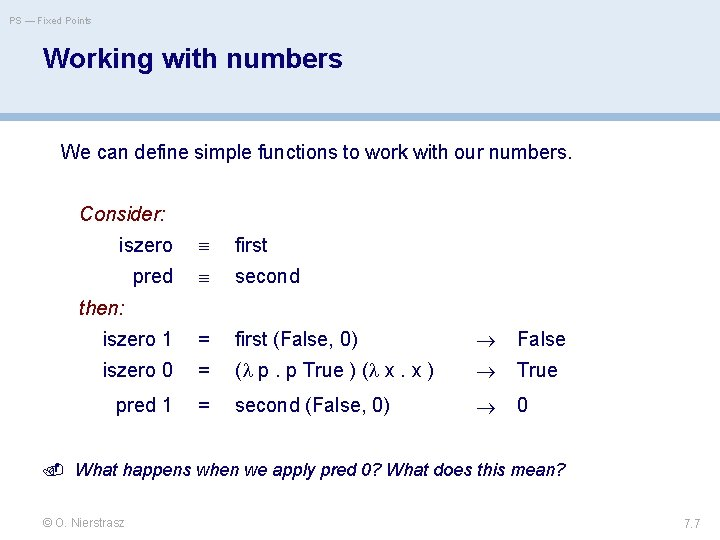PS — Fixed Points Working with numbers We can define simple functions to work with our numbers. Consider: iszero first pred second iszero 1 = first (False, 0) False iszero 0 = ( p. p True ) ( x. x ) True pred 1 = second (False, 0) 0 then: What happens when we apply pred 0? What does this mean? © O. Nierstrasz 7. 7PS — Fixed Points Roadmap > > > Representing Numbers Recursion and the Fixed-Point Combinator The typed lambda calculus The polymorphic lambda calculus Other calculi © O. Nierstrasz 7. 8PS — Fixed Points Recursion Suppose we want to define arithmetic operations on our lambdaencoded numbers. In Haskell we can program: plus n m | n == 0 | otherwise = m = plus (n-1) (m+1) so we might try to “define”: plus n m. iszero n m ( plus ( pred n ) ( succ m ) ) Unfortunately this is not a definition, since we are trying to use plus before it is defined. I. e, plus is free in the “definition”! © O. Nierstrasz 7. 9PS — Fixed Points Recursive functions as fixed points We can obtain a closed expression by abstracting over plus: rplus n m. iszero n m ( plus ( pred n ) ( succ m ) ) rplus takes as its argument the actual plus function to use and returns as its result a definition of that function in terms of itself. In other words, if fplus is the function we want, then: rplus fplus I. e. , we are searching for a fixed point of rplus. . . © O. Nierstrasz 7. 10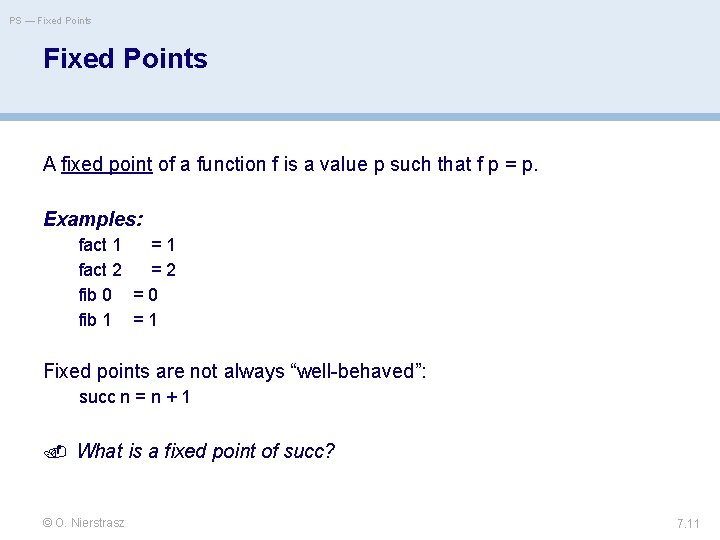PS — Fixed Points A fixed point of a function f is a value p such that f p = p. Examples: fact 1 = 1 fact 2 = 2 fib 0 = 0 fib 1 = 1 Fixed points are not always “well-behaved”: succ n = n + 1 What is a fixed point of succ? © O. Nierstrasz 7. 11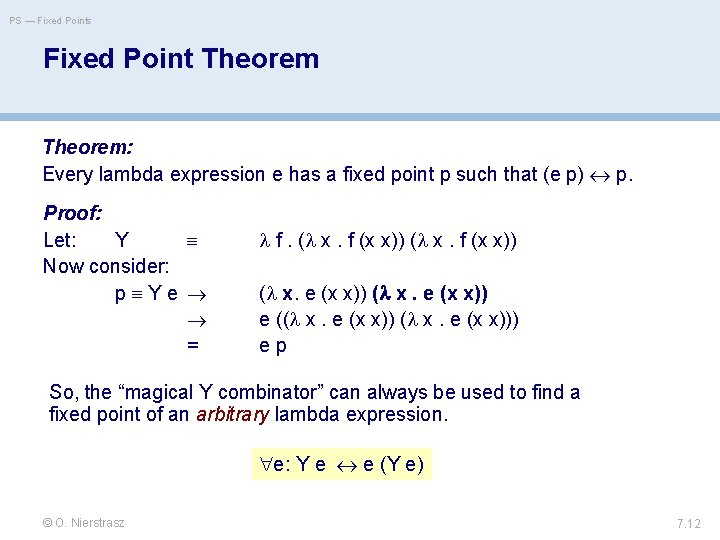PS — Fixed Points Fixed Point Theorem: Every lambda expression e has a fixed point p such that (e p) p. Proof: Let: Y Now consider: p Y e = f. ( x. f (x x)) ( x. e (x x)) e (( x. e (x x))) e p So, the “magical Y combinator” can always be used to find a fixed point of an arbitrary lambda expression. e: Y e e (Y e) © O. Nierstrasz 7. 12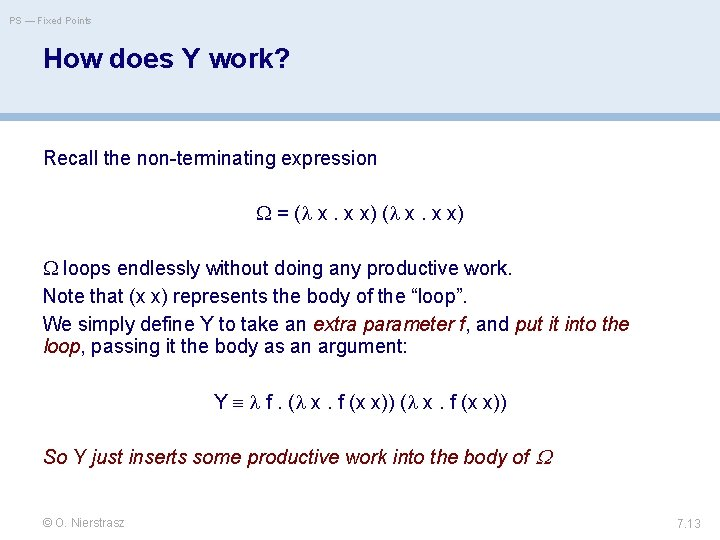PS — Fixed Points How does Y work? Recall the non-terminating expression = ( x. x x) loops endlessly without doing any productive work. Note that (x x) represents the body of the “loop”. We simply define Y to take an extra parameter f, and put it into the loop, passing it the body as an argument: Y f. ( x. f (x x)) So Y just inserts some productive work into the body of © O. Nierstrasz 7. 13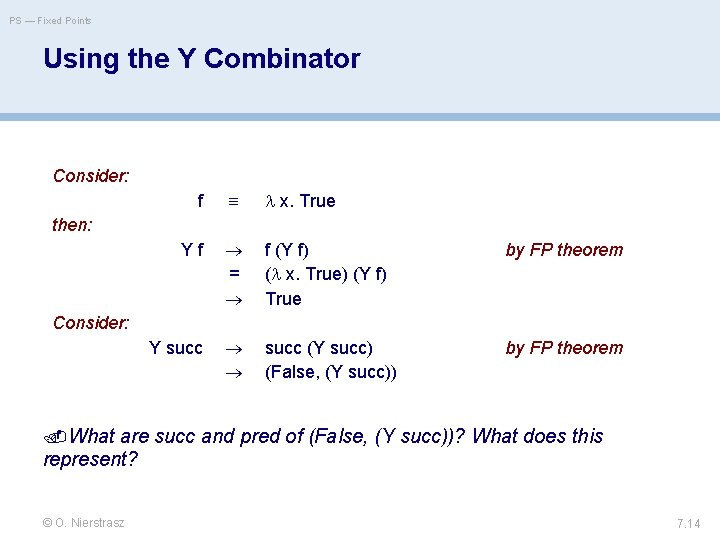PS — Fixed Points Using the Y Combinator Consider: f x. True then: Y f = f (Y f) ( x. True) (Y f) True by FP theorem succ (Y succ) (False, (Y succ)) by FP theorem Consider: Y succ What are succ and pred of (False, (Y succ))? What does this represent? © O. Nierstrasz 7. 14PS — Fixed Points Recursive Functions are Fixed Points We seek a fixed point of: rplus n m. iszero n m ( plus ( pred n ) ( succ m ) ) By the Fixed Point Theorem, we simply take: plus Y rplus Since this guarantees that: rplus as desired! © O. Nierstrasz 7. 15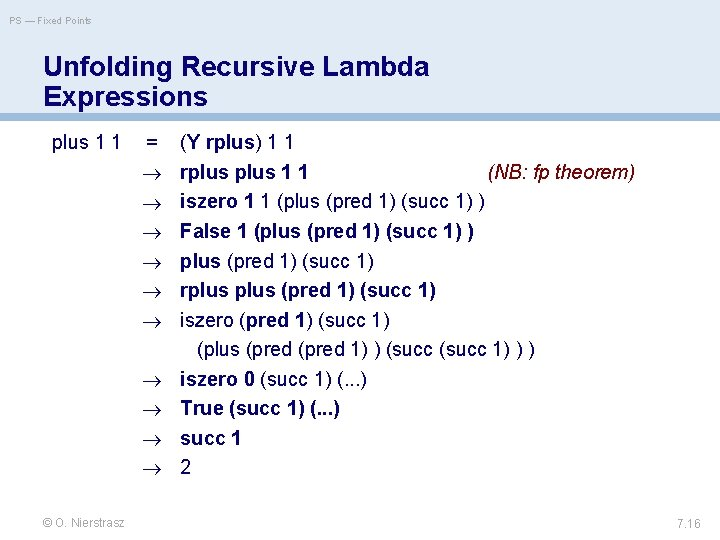PS — Fixed Points Unfolding Recursive Lambda Expressions plus 1 1 = © O. Nierstrasz (Y rplus) 1 1 rplus 1 1 (NB: fp theorem) iszero 1 1 (plus (pred 1) (succ 1) ) False 1 (plus (pred 1) (succ 1) ) plus (pred 1) (succ 1) rplus (pred 1) (succ 1) iszero (pred 1) (succ 1) (plus (pred 1) ) (succ 1) ) ) iszero 0 (succ 1) (. . . ) True (succ 1) (. . . ) succ 1 2 7. 16PS — Fixed Points Roadmap > > > Representing Numbers Recursion and the Fixed-Point Combinator The typed lambda calculus The polymorphic lambda calculus Other calculi © O. Nierstrasz 7. 17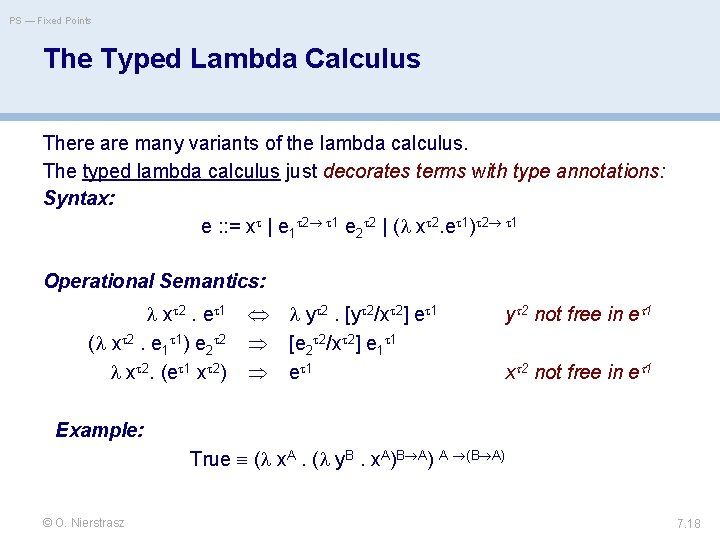PS — Fixed Points The Typed Lambda Calculus There are many variants of the lambda calculus. The typed lambda calculus just decorates terms with type annotations: Syntax: e : : = x | e 1 2 1 e 2 2 | ( x 2. e 1) 2 1 Operational Semantics: x 2. e 1 ( x 2. e 1 1) e 2 2 x 2. (e 1 x 2) y 2. [y 2/x 2] e 1 [e 2 2/x 2] e 1 1 e 1 y 2 not free in e 1 x 2 not free in e 1 Example: True ( x. A. ( y. B. x. A)B A) A (B A) © O. Nierstrasz 7. 18PS — Fixed Points Roadmap > > > Representing Numbers Recursion and the Fixed-Point Combinator The typed lambda calculus The polymorphic lambda calculus Other calculi © O. Nierstrasz 7. 19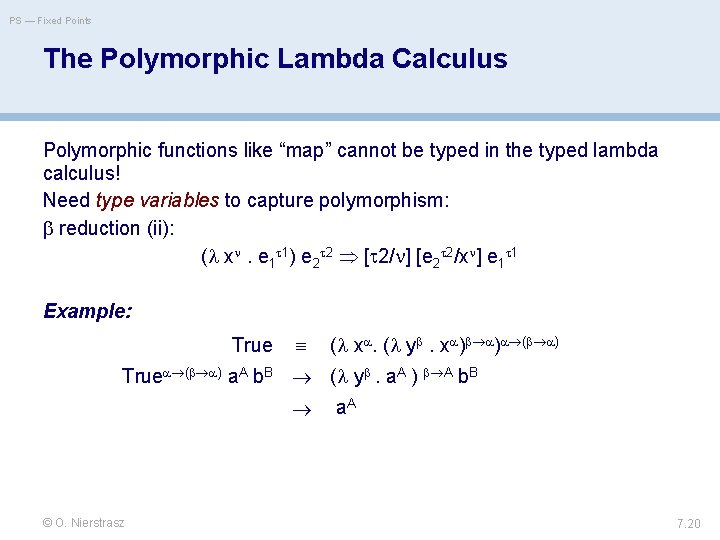PS — Fixed Points The Polymorphic Lambda Calculus Polymorphic functions like “map” cannot be typed in the typed lambda calculus! Need type variables to capture polymorphism: reduction (ii): ( x . e 1 1) e 2 2 [ 2/ ] [e 2 2/x ] e 1 1 Example: True ( x. ( y . x ) ) ( ) True ( ) a. A b. B ( y . a. A ) A b. B a. A © O. Nierstrasz 7. 20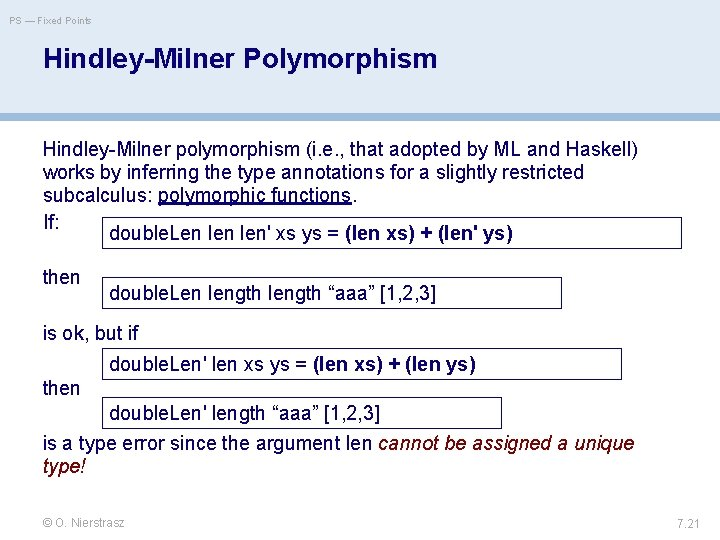PS — Fixed Points Hindley-Milner Polymorphism Hindley-Milner polymorphism (i. e. , that adopted by ML and Haskell) works by inferring the type annotations for a slightly restricted subcalculus: polymorphic functions. If: double. Len len' xs ys = (len xs) + (len' ys) then double. Len length “aaa” [1, 2, 3] is ok, but if double. Len' len xs ys = (len xs) + (len ys) then double. Len' length “aaa” [1, 2, 3] is a type error since the argument len cannot be assigned a unique type! © O. Nierstrasz 7. 21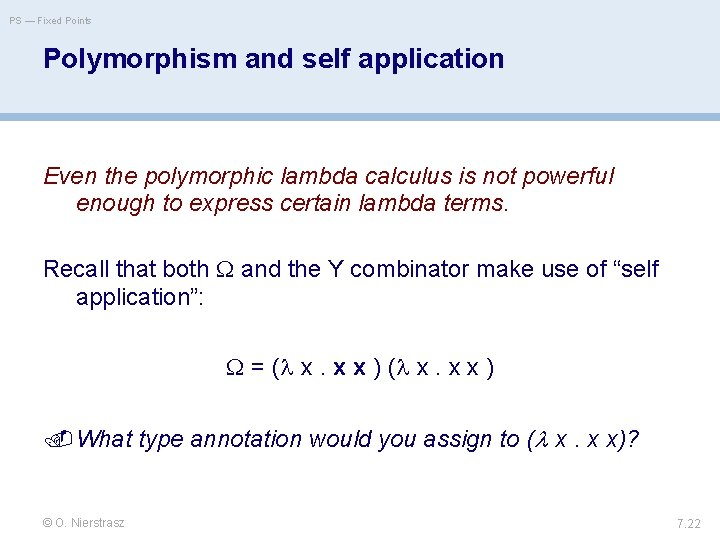PS — Fixed Points Polymorphism and self application Even the polymorphic lambda calculus is not powerful enough to express certain lambda terms. Recall that both and the Y combinator make use of “self application”: = ( x. x x ) What type annotation would you assign to ( x. x x)? © O. Nierstrasz 7. 22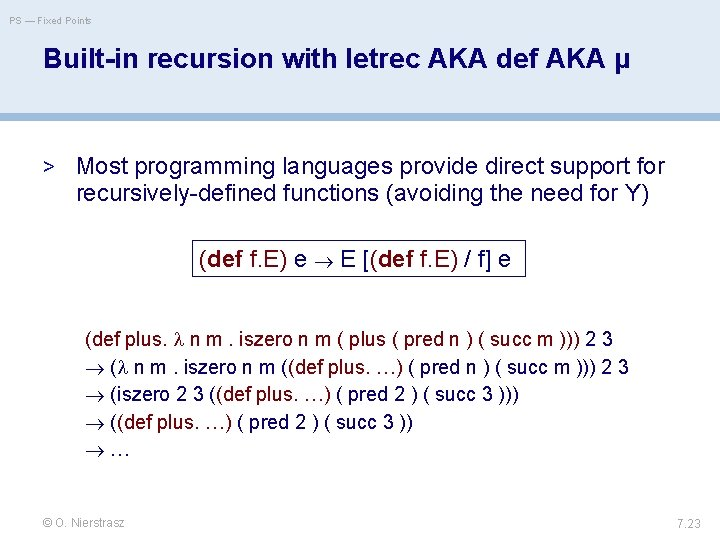PS — Fixed Points Built-in recursion with letrec AKA def AKA µ > Most programming languages provide direct support for recursively-defined functions (avoiding the need for Y) (def f. E) e E [(def f. E) / f] e (def plus. n m. iszero n m ( plus ( pred n ) ( succ m ))) 2 3 ( n m. iszero n m ((def plus. …) ( pred n ) ( succ m ))) 2 3 (iszero 2 3 ((def plus. …) ( pred 2 ) ( succ 3 ))) ((def plus. …) ( pred 2 ) ( succ 3 )) … © O. Nierstrasz 7. 23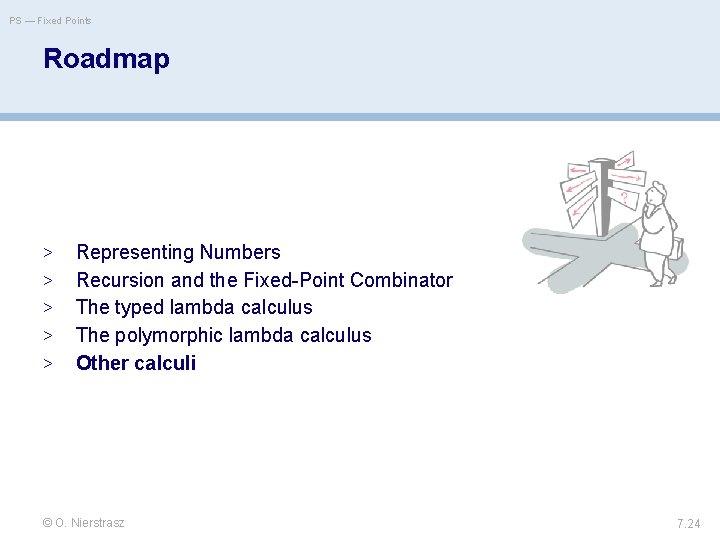PS — Fixed Points Roadmap > > > Representing Numbers Recursion and the Fixed-Point Combinator The typed lambda calculus The polymorphic lambda calculus Other calculi © O. Nierstrasz 7. 24PS — Fixed Points Featherweight Java Used to prove that generics could be added to Java without breaking the type system. Igarashi, Pierce and Wadler, “Featherweight Java: a minimal core calculus for Java and GJ”, OOPSLA ’ 99 doi. acm. org/10. 1145/320384. 320395 © O. Nierstrasz 7. 25PS — Fixed Points Other Calculi Many calculi have been developed to study the semantics of programming languages. Object calculi: model inheritance and subtyping. . — lambda calculi with records Process calculi: model concurrency and communication — CSP, CCS, pi calculus, CHAM, blue calculus Distributed calculi: model location and failure — ambients, join calculus © O. Nierstrasz 7. 26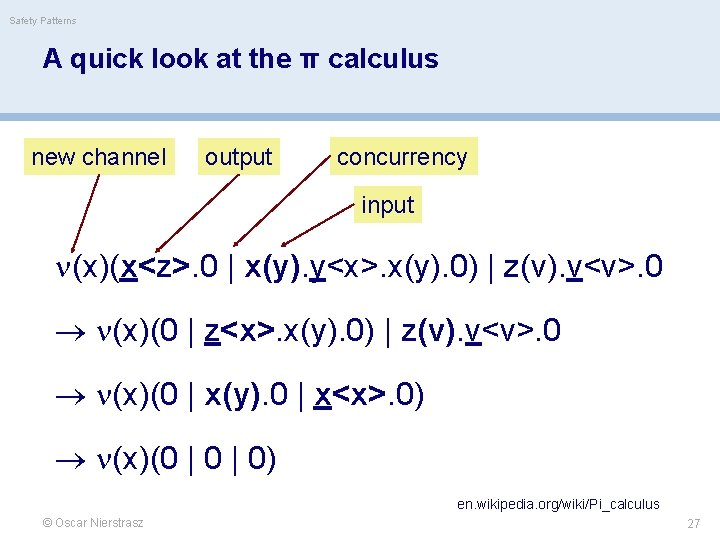Safety Patterns A quick look at the π calculus new channel output concurrency input (x)(x<z>. 0 | x(y). y<x>. x(y). 0) | z(v). v<v>. 0 (x)(0 | z<x>. x(y). 0) | z(v). v<v>. 0 (x)(0 | x(y). 0 | x<x>. 0) (x)(0 | 0) en. wikipedia. org/wiki/Pi_calculus © Oscar Nierstrasz 27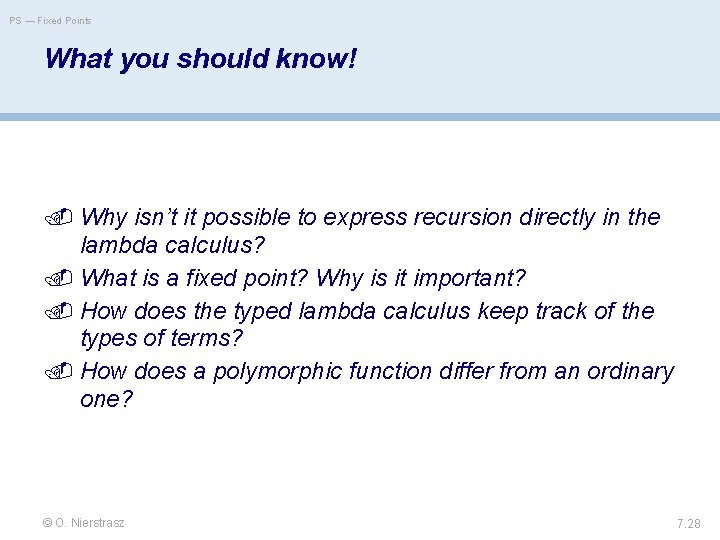PS — Fixed Points What you should know! Why isn’t it possible to express recursion directly in the lambda calculus? What is a fixed point? Why is it important? How does the typed lambda calculus keep track of the types of terms? How does a polymorphic function differ from an ordinary one? © O. Nierstrasz 7. 28PS — Fixed Points Can you answer these questions? How would you model negative integers in the lambda calculus? Fractions? Is it possible to model real numbers? Why, or why not? Are there more fixed-point operators other than Y? How can you be sure that unfolding a recursive expression will terminate? Would a process calculus be Church-Rosser? © O. Nierstrasz 7. 29